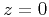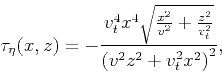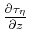Traveltime approximations for transversely isotropic media with an inhomogeneous backgroundNext: Appendix D: Expansion in Up: Alkhalifah: TI traveltimes in Previous: Appendix B: Expansion in

# Appendix C: The homogeneous medium case

To develop analytical traveltime representation for TI media, I start with a background velocity model that is homogeneous. The expansion here will be with respect toandfrom a background elliptical anisotropic model. In this case, the traveltime from a point source atandis given by the following simple relation in 2-D:(39)

which satisfies the eikonal equation B-2. Using equation C-1, I evaluateandand insert them into equation B-3 to solve the first-order linear equation to obtain(40)

as well as insert them into equation B-4 and solve the equation to obtain(41)

I now evaluateandand use them to solve equation B-5. After some tedious algebra, I obtain(42)

I also evaluateandand use them to solve equation B-6 to obtain(43)

Finally, I solve equation B-7. After some tedious algebra once again, I obtain(44)

Using the first sequence of Shanks transform, equation B-9, applied to the Taylor's series expansion, we obtain an analytical equation that describes traveltime as a function ofand.

For 3-D media, I include the azimuth angle as we will see next.Traveltime approximations for transversely isotropic media with an inhomogeneous backgroundNext: Appendix D: Expansion in Up: Alkhalifah: TI traveltimes in Previous: Appendix B: Expansion in

2013-04-02